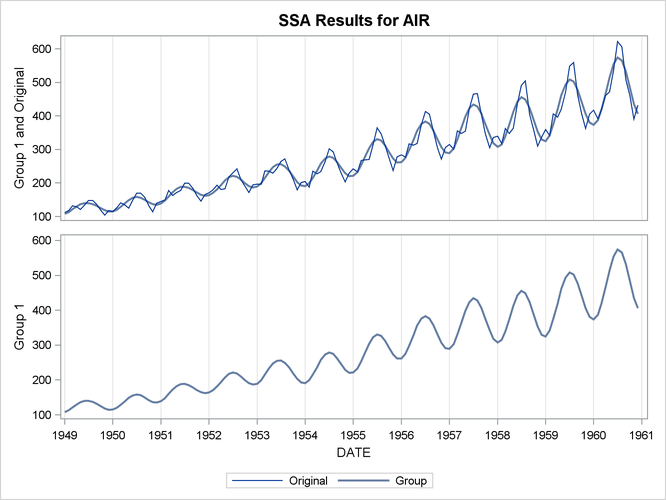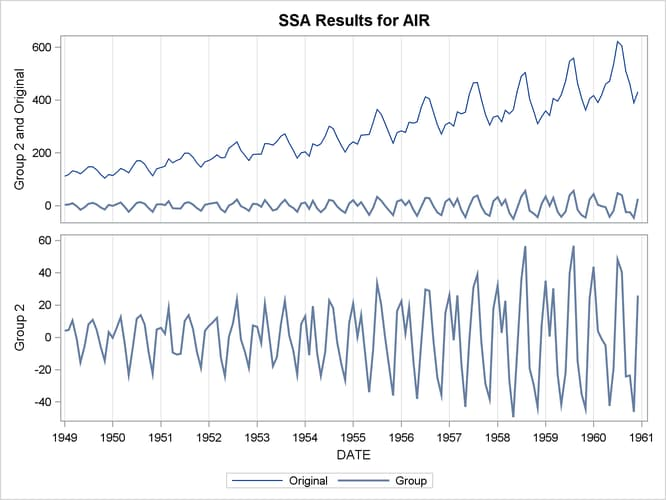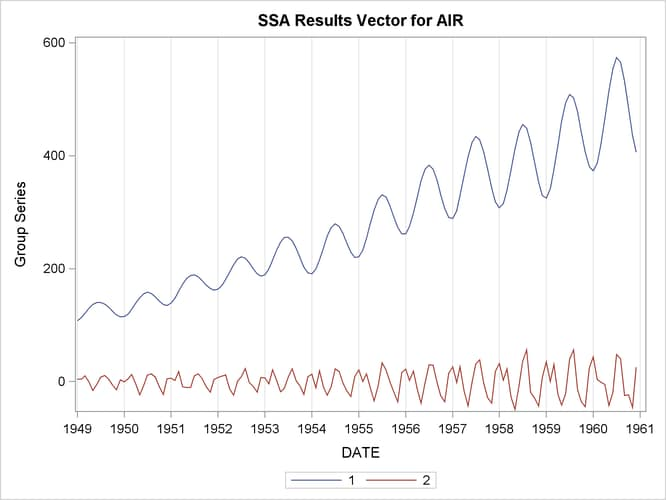The TIMESERIES Procedure

Example 32.5 Illustration of Singular Spectrum Analysis

This example illustrates the use of singular spectrum analysis.

The following statements extract two additive components from the `SASHELP.AIR` time series by using the THRESHOLDPCT= option to specify that the first component represent 80% of the variability in the series. The resulting groupings, consisting of the first three and remaining nine singular value components, are presented in Output 32.5.1 through Output 32.5.3.

```title "SSA of AIR data";

proc timeseries data=sashelp.air plot=ssa;
id date interval=month;
var air;
ssa / length=12 THRESHOLDPCT=80;
run;
```

Output 32.5.1: Singular Value Grouping #1 PlotOutput 32.5.2: Singular Value Grouping #2 PlotOutput 32.5.3: Singular Value Components Plot